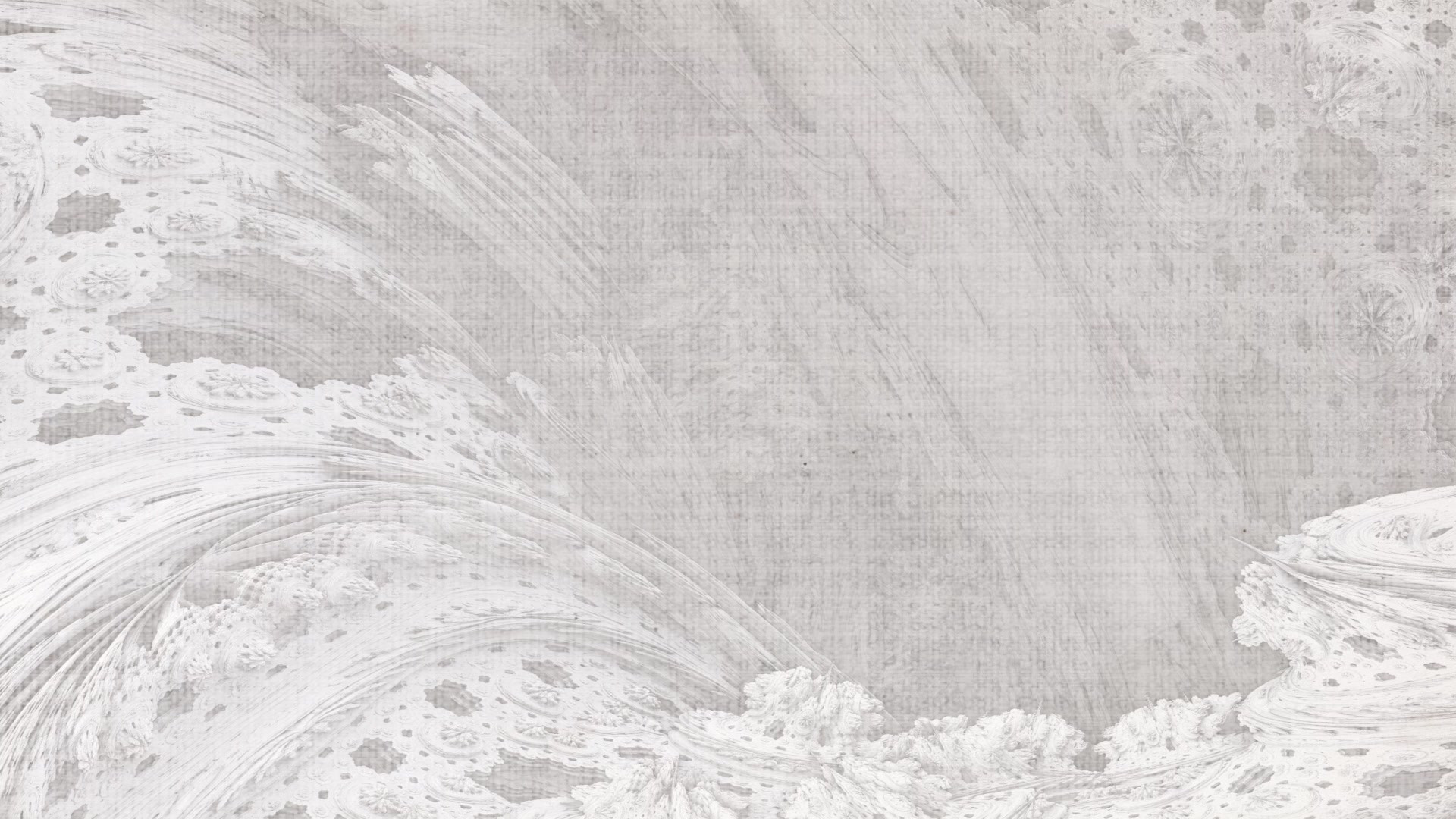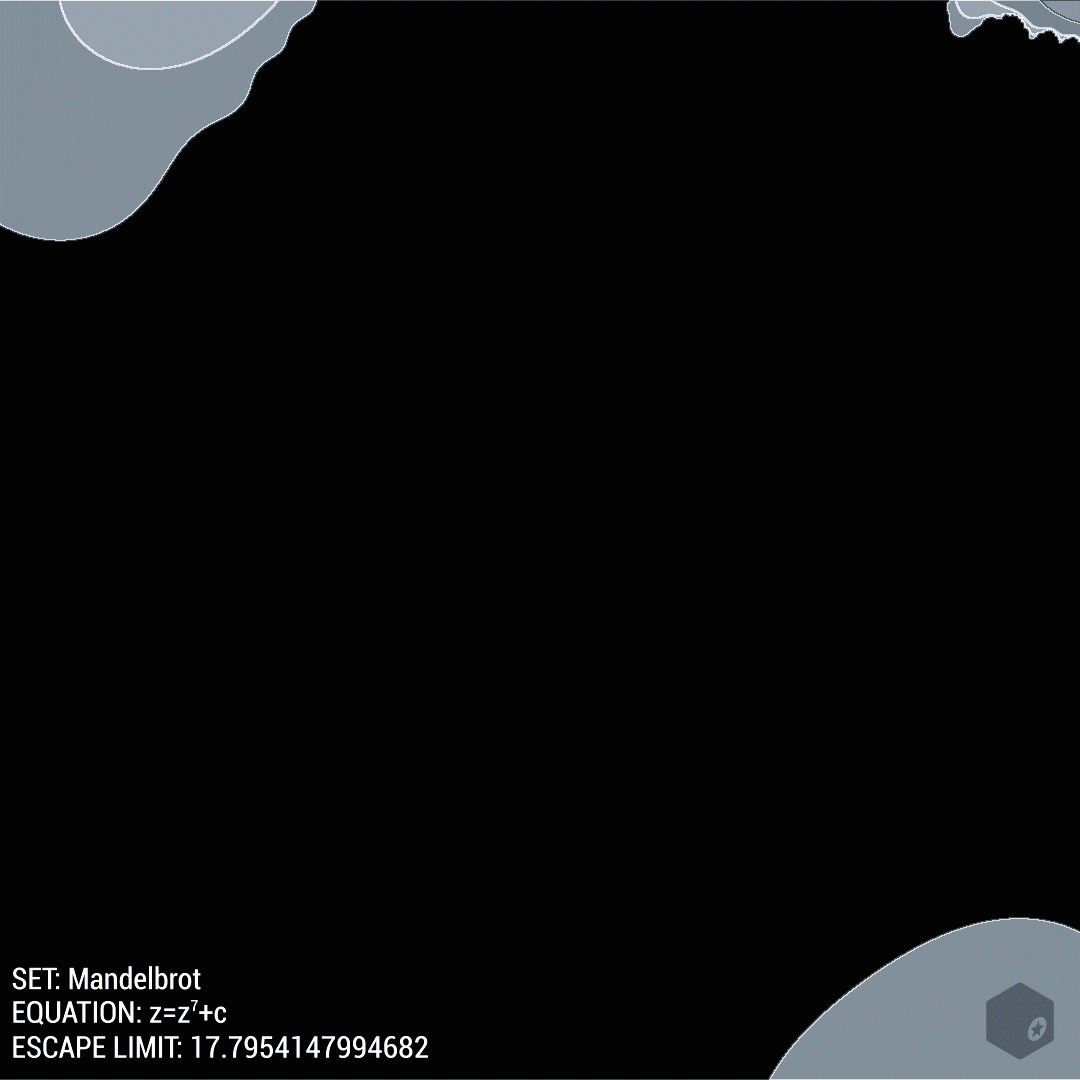## DEFINITION

frac·tal

ˈfraktəl/

MATHEMATICS

noun

1. a curve or geometric figure, each part of which has the same statistical character as the whole. Fractals are useful in modeling structures (such as eroded coastlines or snowflakes) in which similar patterns recur at progressively smaller scales, and in describing partly random or chaotic phenomena such as crystal growth, fluid turbulence, and galaxy formation.

1. 1.relating to or of the nature of a fractal or fractals.

2. "fractal geometry"

confusing

1. a geometrical or physical structure having an irregular or fragmented shape at all scales of measurement between a greatest and smallest scale such that certain mathematical or physical properties of the structure, as the perimeter of a curve or the flow rate in a porous medium, behave as if the dimensions of the structure (fractal dimensions) are greater than the spatial dimensions.

n8

1. A geometric shape that repeats patterns through orders of magnitude.

dictionary.com

## OVERVIEW

The simplest example of a fractal to visualize is to draw a straight line. Draw two more lines branching from the first in a Y shape at half the length. Do this to the end of every line until you get bored, and you have just barely started making a fractal.

Fractals can be seen in nature in an array of manifestations. The spiral of a snail shell, the recursive diamonds of a sunflower, and the hexagonal crystal patterns of pyrite. All of these share a distinct trait of repeating a recognizable pattern at multiple scales.

CLICK  to focusMANDELBROT z7 2D FRACTAL ITERATIONS

CLICK to zoomMANDELBROT z7 2D FRACTAL EXTENTS

## MODERN DEVELOPMENT

One of the earliest applications of fractals came about well before the term was even used. Lewis Fry Richardson was an English mathematician in the early 20th century studying the length of the English coastline. He reasoned that the length of a coastline depends on the length of the measurement tool. Measure with a yardstick, you get one number, but measure with a more detailed foot-long ruler, which takes into account more of the coastline's irregularity, and you get a larger number, and so on.

Carry this to its logical conclusion and you end up with an infinitely long coastline containing a finite space, the same paradox put forward by Helge von Koch in the Koch Snowflake. This fractal involves taking a triangle and turning the central third of each segment into a triangular bump in a way that makes the fractal symmetric. Each bump is, of course, longer than the original segment, yet still contains the finite space within. Weird, but rather than converging on a particular number, the perimeter moves towards infinity. Mandelbrot saw this and used this example to explore the concept of fractal dimension, along the way proving that measuring a coastline is an exercise in approximation.

Unconventional 20th century mathematician Benoit Mandelbrot created the term fractal from the Latin word fractus (meaning irregular or fragmented) in 1975.

Benoit Mandelbrot is considered to be the father of contemporary fractal mathematics. He went on to write several books on fractals, their mathematics, and appearance in nature.

CLICK  to focusFRACTAL ITERATION PROGRESSION

CLICK  to pauseFRACTAL MAGNIFICATION DEPTH 2-71, ITERATIONS 6-50